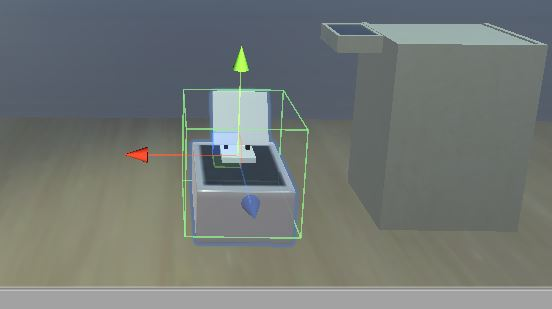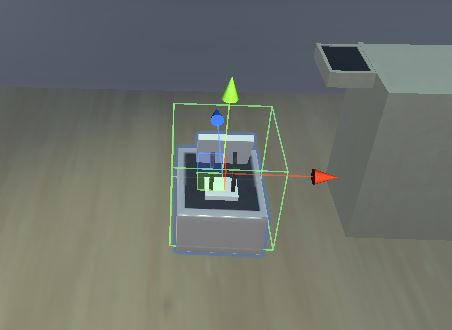2020-04-10 22:53

# Unity 使物体以一定的速度旋转特定的角度

``````void Update(){
if (Input.GetMouseButton(0))
{
Quaternion quater = Quaternion.Euler(0, transform.rotation.y + 90, 0);
transform.rotation = Quaternion.Slerp(transform.rotation,quater, rotateSpeed * Time.deltaTime);
if (Quaternion.Angle(quater, transform.rotation) < 1)
{
transform.rotation = quater;
}
}
}
````````````Quaternion quater = Quaternion.Euler(0, transform.rotation.y + 90, 0);

Quaternion quater = Quaternion.Euler(0, transform.rotation.y + x);

……
Quaternion quater = Quaternion.Euler(0, transform.rotation.y + x);

transform.rotation = Quaternion.Slerp(transform.rotation,quater, rotateSpeed * Time.deltaTime);

x=0;
……

``````